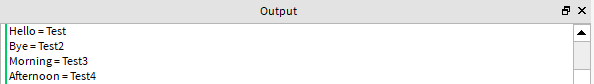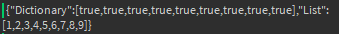# How do I find similar values in arrays?

Hello!

I’m working on a ModuleScript that has many utility functions.
I would like to be able to make an array of values all my arrays have (more than 2).

In which way could I achieve this?

There may be a more efficient way of doing this however this is how I would combine two tables (as I suppose that’s what you are trying todo):

``````local Table1 = {
Hello = "Test",
Bye = "Test2"
}

local Table2 = {
Morning = "Test3",
Afternoon = "Test4"
}

local TablesToCombine = {Table1, Table2}
local CombinedTable = {}

for i,Table in ipairs(TablesToCombine) do
for i,v in pairs(Table) do
CombinedTable[i] = v
end
end

--Testing Result
for i,v in pairs(CombinedTable) do
print(i.." = "..v)
end
``````3 Likes

You aren’t very specific in your post, what values are you referring to?

If you’re referring to the indices or functions of your Module, you can use a pairs loop.

``````for index, value in pairs(module) do
print(index,value);
end
``````

Sorry I wasn’t very specific,
What I meant was something like this
Array1 = {1,2,3}
Array2 = {1,4,3,9}
MagicFunction(Array1, Array2)
– Returns {1,3}

I’m extremely tired, but here’s my terrible solution.

``````
local function SharedValues(Array1, Array2)
local Dictionary1 = {}
for _, Value in ipairs(Array1) do
if not Dictionary1[Value] then
Dictionary1[Value] = true
end
end

local Dictionary2 = {}
for _, Value in ipairs(Array2) do
if not Dictionary2[Value] then
Dictionary2[Value] = true
end
end

local Array = {}
local Length = 0
for Value in pairs(Dictionary1) do
if Dictionary2[Value] then
Length = Length + 1
Array[Length] = Value
end
end

return Array
end
``````
3 Likes

(Just a note, you can change your question to how do I check if two tables have some similar values! You might even call it the intersection of two arrays, if they have two similar values.)

A way of doing it is, looping through the first table, and for each value of the first table we loop through the second table to see if there is a value that’s the same. If the values are the same, we insert that value into another table and return that table.

``````local table1 = {1,2,3}
local table2 = {3,4,7,1}

local function Intersection(t1, t2)
local output = {}
for _, v in pairs(t1) do
for __, h in pairs(t2) do --we gotta use a different name other than "v" of course
if v == t then
table.insert(output, v)
end
end
end
return output
end
``````

You can even use the method @howmanysmaII provided which uses dictionarries instead!
By doing

``````if table1[value] then
``````

We are basically checking if that value exists, `table1[value]` will give back a value (whatever the value is) and since any value other then false or nil is truthy, the if statment will pass. If the value didn’t exit, which means it will return `nil`, the if statments wouldn’t pass

1 Like

Just so you know, you don’t need to do `__` in a later variable. It’s already ignoring the `_` from before.

2 Likes

Are you sure this is a bad solution?

It’s not the best I could do if I had any sleep (O(n) isn’t ideal), but it’s alright.

1 Like

Are you sure its not the best you can do?
Looks good to me

``````function MultiArrayValueMatch(...)
local ArrayList  = {...}
local matches = {}
for _, Array in pairs(ArrayList) do
if typeof(Array) == "table" then
for i, v in pairs(Array) do
if matches[v] == nil then
matches[v] = true
end
end
end
end
local Result = {}
for Key, _ in pairs(matches) do
Result[#Result+1] = Key
end
return {Dictionary = matches, List = Result}
end
local MatchedList = MultiArrayValueMatch({1,2,3,4}, {3,4,5,6,7}, {6,7,8,9}, {10,11,12,1,2})
``````

You could use this function to check as many tables as you like and it will give you whichever format you wish, List to get something like {1,2,3} or Dictionary to get { = true,  = true,  = true} so you can later do something like

``````if MatchedList[NumberDesiredHere]  then

end
``````
3 Likes

It’s fine, it’s just not ideal.

1 Like

The function you provided seems to be merging the array and putting true for every valueTested with: {1,2,3,4,5,6},{6,7},{6,8,9}

1 Like

how are you trying to output it @noahcoolboy2

I use print(game.HttpService:JSONEncode(“TableHere”))
I Can clearly see the table like this

``````function MultiArrayValueMatch(...)
local ArrayList  = {...}
local matches = {}
for _, Array in pairs(ArrayList) do
if typeof(Array) == "table" then
for i, v in pairs(Array) do
if matches[v] == nil then
matches[v] = true
end
end
end
end
local Result = {}
for Key, _ in pairs(matches) do
Result[#Result+1] = Key
end
return {Dictionairy = matches, List = Result}
end
local MatchedList = MultiArrayValueMatch({1,2,3,4}, {3,4,5,6,7}, {6,7,8,9}, {10,11,12,1,2})
for ListKey, _ in pairs(MatchedList.Dictionairy) do
print("Dictionairy key:", ListKey)
end
for _, Num in ipairs(MatchedList.List) do
print("List value:", Num)
end
``````

The JSON string doesn’t really help in that matter because the keys in that “dictionary” are numbers soo you could do this:

``````function MultiArrayValueMatch(...)
local ArrayList  = {...}
local matches = {}
for _, Array in pairs(ArrayList) do
if typeof(Array) == "table" then
for i, v in pairs(Array) do
if matches[tostring(v)] == nil then
matches[tostring(v)] = true
end
end
end
end
local Result = {}
for Key, _ in pairs(matches) do
Result[#Result+1] = Key
end
return {Dictionairy = matches, List = Result}
end
local MatchedList = MultiArrayValueMatch({1,2,3,4}, {3,4,5,6,7}, {6,7,8,9}, {10,11,12,1,2})
print(game:GetService("HttpService"):JSONEncode(MatchedList.Dictionairy))
``````

Actually you can make it even shorter and do this

``````function MultiArrayValueMatch(...)
local ArrayList  = {...}
local matches,Result = {}, {}
for _, Array in pairs(ArrayList) do
if typeof(Array) == "table" then
for i, v in pairs(Array) do
if matches[tostring(v)] == nil then
matches[tostring(v)] = true
Result[#Result+1] = v
end
end
end
end
return {Dictionary = matches, List = Result}
end
local MatchedList = MultiArrayValueMatch({1,2,3,4}, {3,4,5,6,7}, {6,7,8,9}, {10,11,12,1,2})
print(game:GetService("HttpService"):JSONEncode(MatchedList.List))
``````

@noahcoolboy2

1 Like

Probably not the most efficient

``````function IndexOf(Array,Value,FromIndex)
Array = Array or {}
Value = Value or nil
FromIndex = FromIndex or 1
for i,v in ipairs(Array) do
if v == Value and i >= FromIndex then
return i
end
end
return -1
end
function MultiArrayMatch(...)
local ArrayList  = {...}
local Result = {}
for i,v in pairs(ArrayList) do
for i,o in pairs(v) do
Result[o] = true
end
end
for i,v in pairs(ArrayList) do
for i,o in pairs(Result) do
if IndexOf(v, i) == -1 then
Result[i] = nil
end
end
end
return Result
end

MultiArrayMatch({1,2,3,4,5,6},{6,7},{6,8,9})``````
2 Likes